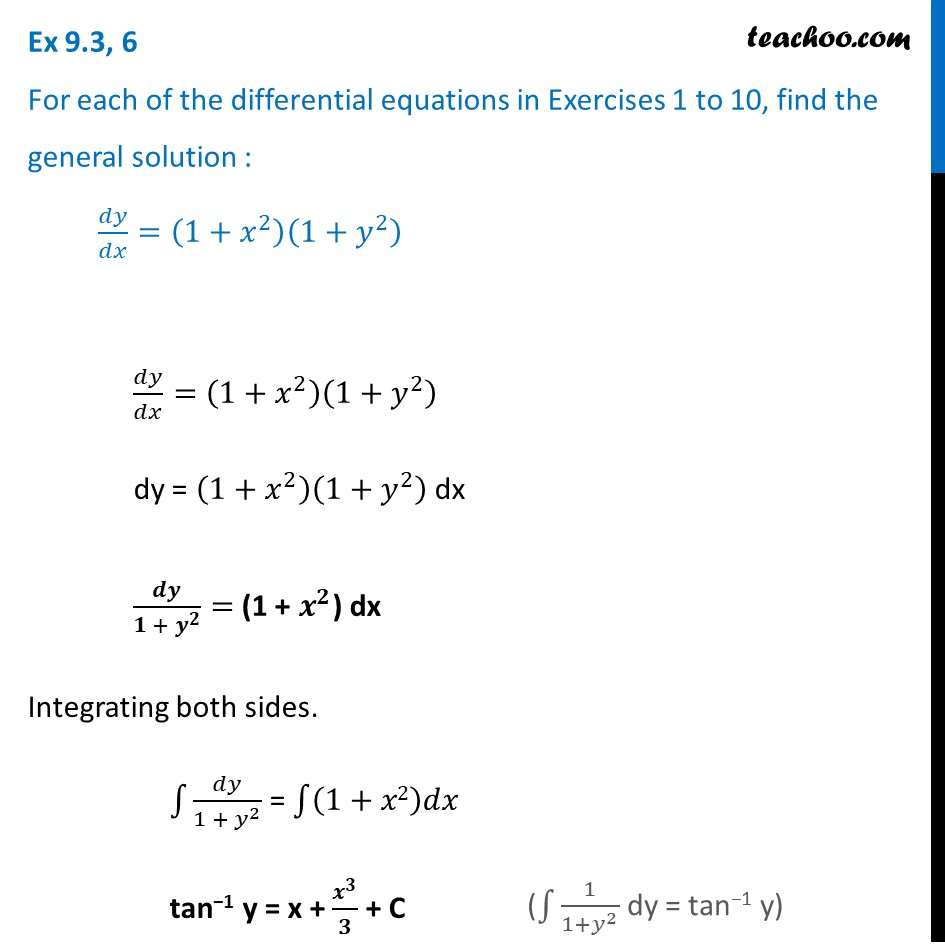Ex 9.3

Chapter 9 Class 12 Differential Equations
Serial order wiseLearn in your speed, with individual attention - Teachoo Maths 1-on-1 Class

### Transcript

Ex 9.3, 6 For each of the differential equations in Exercises 1 to 10, find the general solution : 𝑑𝑦/𝑑𝑥=(1+𝑥^2 )(1+𝑦^2 )𝑑𝑦/𝑑𝑥=(1+𝑥^2 )(1+𝑦^2 ) dy = (1+𝑥^2 )(1+𝑦^2 ) dx 𝒅𝒚/(𝟏 + 𝒚^𝟐 )= (1 + 𝒙^𝟐) dx Integrating both sides. ∫1▒𝑑𝑦/(1 + 𝑦^2 ) = ∫1▒(1+𝑥2)𝑑𝑥 tan−1 y = x + 𝒙^𝟑/𝟑 + C (∫1▒1/(1+𝑦^2 ) dy = tan−1 y)